# How to Paste Range Names in Excel

This tutorial demonstrates how to paste range names in Excel.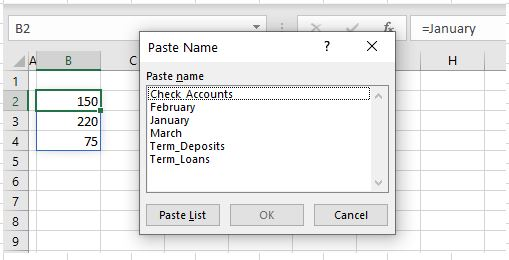If you have a workbook that contains a lot of named ranges, you can use those range names in alternative locations or formulas in your Excel file.

## Paste Range Name to Another Location

1. First, select the cell where you want to paste the range name.
2. In the Ribbon, go to Formulas > Defined Names > Use in Formula > Paste Names…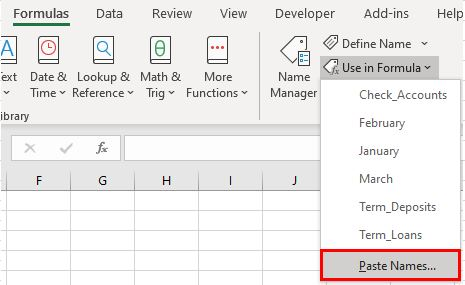1. Select the name you want to paste, then click OK.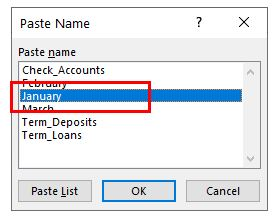1. Press the ENTER key on the keyboard or click the check mark in the formula bar.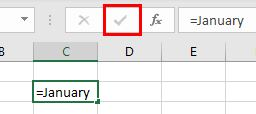The range name is inserted as a dynamic array. You cannot edit individual cells in a dynamic array or delete the values in the cells underneath your formula (for example the values in C3 and C4 in the example below): The cells, together, form part of an individual range name.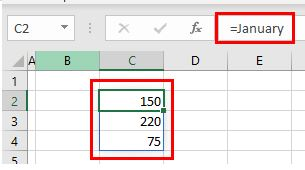## Paste Range Name Into a Formula

You can also paste range names into formulas.

1. First, type the beginning of the formula. (This example uses the SUM Function.)
2. In the Ribbon, go to Formulas > Defined Names > Use in Formula > Paste Names… Then choose the name you want and click OK.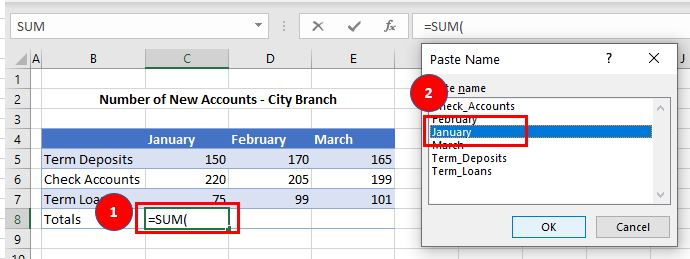1. Press ENTER for Excel to automatically complete the formula with a closing parenthesis.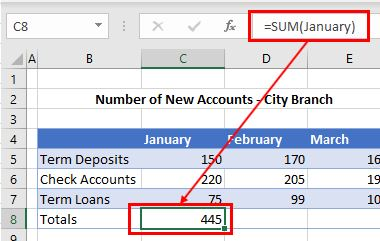### Use Name in Formula List

An alternative (and simpler!) way to paste a range name into a formula is to directly select the name from the Use in Formula list.

1. Type the first part of the formula.
2. In the Ribbon, go to Formulas > Defined Names > Use in Formula, then click the name you need.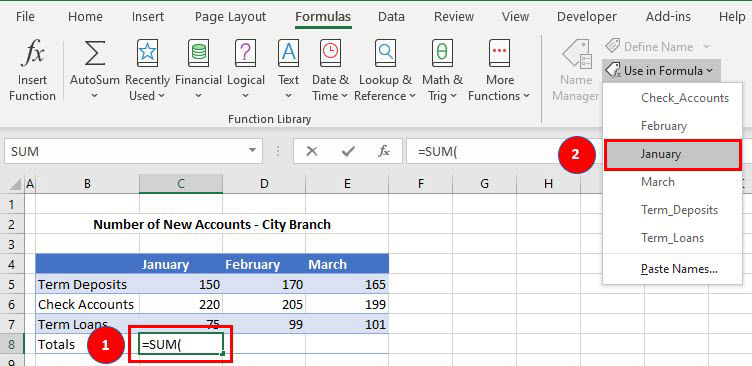1. Press ENTER for Excel to automatically complete the formula with a closing parenthesis.